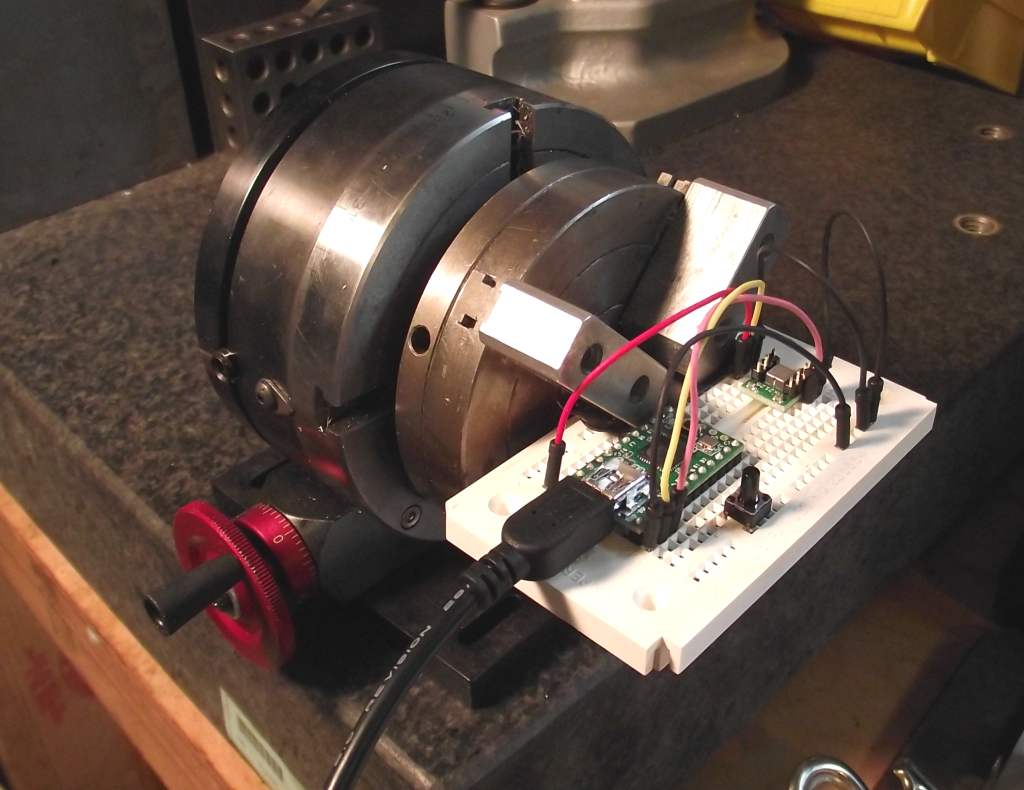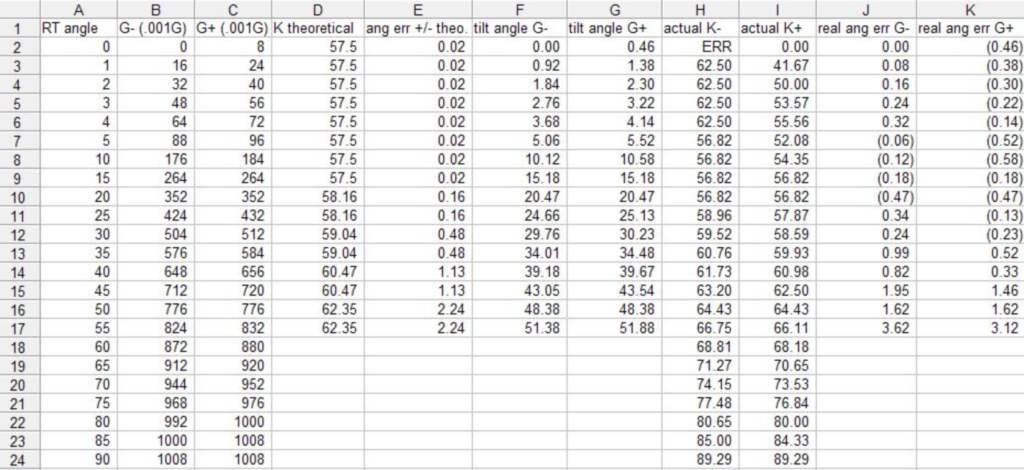# Parallax Memsic 2125 Angle Test

I picked up one of the Parallax Memsic 2125 accelerometers at Radio Shack this weekend (because they had a nice coupon for \$15 off a \$50 purchase). I was wondering how accurate it was in terms of angular measurment so I rigged up a Sherline rotary table to hold my breadboard with a Teensy 2.0 and the sensor and measured the figures for G (.001 G) for angles from 0 to 5 by one degree and by 5 up to 90 degrees.This is the code: void setup() { pinMode(0, INPUT); pinMode(1, INPUT); pinMode(PIN_D0, INPUT_PULLUP); // Pushbutton Serial.begin(9600); }

void loop() { // variables to read the pulse widths: int pulseX, pulseY; // variables to contain the resulting accelerations int accelerationX, accelerationY;

// read pulse from x- and y-axes: pulseX = pulseIn(0,HIGH); pulseY = pulseIn(1,HIGH);

// convert the pulse width into acceleration // accelerationX and accelerationY are in milli-g's: // earth's gravity is 1000 milli-g's, or 1g. accelerationX = ((pulseX / 10) - 500) * 8; accelerationY = ((pulseY / 10) - 500) * 8;

Serial.println("X axis"); Serial.print(accelerationX);

delay(1000); }

I looked up the Parallax data on it and found this .PDF: http://www.parallax.com/Portals/0/Downloads/docs/cols/nv/vol3/col/nv92.pdf

It gives a table that uses a constant k for various angles from +/-10 deg. to +/-50 deg. Where the angle = g x k

I found that my G (acceleration) measurements wiggled between two values, probably due to a host of reasons. So I computed for the upper and lower figure for each angle on the rotary table. 0 degrees is where I started from when the accelerometer read between 0 and 8. I could not get it to settle down on 0.This is all a bit confusing, but taking 45 degrees as an example, the value of G varied between 712 and 720. The theortical constant is 60.47, but the actual constant was between 63.2 and 62.5, and the error of the theoretical angle vs. the actual angle using the table's value of K was between 1.95 and 1.46 degrees. The error gets greater the more extreme the angle is from horizontal. I'm sure that my rotary table setup contributed partly to the error, and measurements probably are on the order of +/-.1 degrees, however being on the granite plate I didn't notice that vibrations (such as me pounding on my bench) effected the readings. I'm going to try using float instead of int for the variables and see if I can wring a little more precision from the code as well as looking at the raw pulse length value for various angles. I will probably have to make a table of my own constant (k) and use that in any code that I want to output an angle. Anyway, this was a fun little test. (and my first on topic Arduino forum post!)